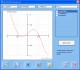DeadLine 2.36 Analyze, solve, and plot various mathematical equations. Freeware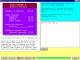Basic Algebra Shape-Up 4.0 Hands-on algebra tutorials covering integers, expressions, equations, and more. Shareware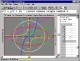Graphmatica 2.4 powerful, easy-to-use, equation plotter with numerical and calculus features Shareware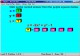Algebra - One On One 1.1 Makes learning algebra fun. 21 skill levels. Learn step by step. Shareware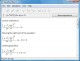Equation Wizard 1.21 Automatically solves algebraic equations of any order written in any form SharewarePDF Equation 0.7 PDF Equation gives you quick access to LaTeX's typesetting power in almost every Mac OS X application. FreewareSudoku Online Solver 1.2 Sudoku Online Solver FreewareEquation Service 1.0 Equation Service is a program that uses pdflatex to produce small PDF files containing equations and other text. FreewareChemical Equation Expert 2.12a Chemical Equation Expert is an integrated tool for chemistry professionals Freeware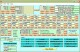Linear Algebra 2 Performs computations associated with matrices. Shareware